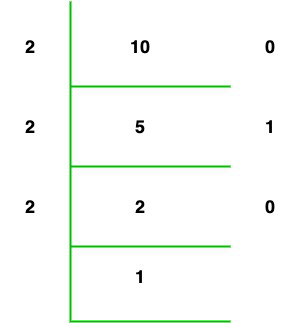Open In App

# C# Program to Find Binary Equivalent of an Integer using Recursion

Given an integer number, now we convert the given integer number into a binary number using recursion. Recursion is a method in which a function calls itself directly or indirectly and such type of function is known as a recursive function. It solves the problem very efficiently like we find the binary equivalent of an integer.

Examples:

```Input : 10
Output: 1010

Input : 11
Output: 1011```

Approach:

To display the binary equivalent of an integer we use the following steps:

• If condition is used to check if the given value is not equal to zero.
• If the given condition is true then perform the modulus of the val by 2, then add the modulus result to 10 and then multiply the value of the result with the value of decimaltobinary() function.
• Now repeat step 2 until the value of val variable is greater than zero.
• Print the array in reverse order now.
• And if the condition is false then it will execute the else section, i.e., return 0Let us considered the integer number is 10. Now we find the binary equivalent of 10 so,

• 10 % 2 + 10 * (10 / 2) % 2 will return 0
• 5 % 2 + 10 * (5 / 2) % 2 will return 1
• 2 % 2 + 10 * (2 / 2) % 2 will return 0
• 1 % 2 + 10 * (1 / 2) % 2 will return 1

So the final result is 1010.

Example 1:

## C#

 `// C# program to display the binary equivalent``// of an integer``using` `System;`` ` `class` `GFG{`` ` `// Driver code``public` `static` `void` `Main(``string``[] args)``{``     ` `    ``// Input``    ``int` `num = 15;``     ` `    ``decimaltobinary(num);``}`` ` `// Function to display the binary equivalent ``// of an integer``public` `static` `int` `decimaltobinary(``int` `val)``{``    ``int` `binary;``     ` `    ``if` `(val != 0)``    ``{``        ``binary = (val % 2) + 10 * decimaltobinary(val / 2);``        ``Console.Write(binary);``        ``return` `0;``    ``}``    ``else``    ``{``        ``return` `0;``    ``}``}``}`

Output

`1111`

Example 2:

## C#

 `// C# program to display the binary equivalent``// of an integer``using` `System;`` ` `class` `GFG{``   ` `// Function to display the binary equivalent ``// of an integer``public` `static` `int` `decimaltobinary(``int` `val)``{``    ``int` `binary;``     ` `    ``if` `(val != 0)``    ``{``        ``binary = (val % 2) + 10 * decimaltobinary(val / 2);``        ``Console.Write(binary);``        ``return` `0;``    ``}``    ``else``    ``{``        ``return` `0;``    ``}``}``   ` `// Driver code``public` `static` `void` `Main(``string``[] args)``{``    ``int` `num;``     ` `    ``// Reading input from user``    ``Console.Write(``"Hi! Enter the number:"``);``    ``num = ``int``.Parse(Console.ReadLine());``    ``decimaltobinary(num);`` ` `}``}`

Output:

```Hi! Enter the number:10
1010```